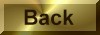Practice Problem 2:

Classify each of the following as either a metathesis or an oxidation-reduction reaction.  Note that mercury usually exists in one of three oxidation states: mercury metal, Hg22+ ions, or Hg2+ ions.

(a) Hg22+(aq) + 2 OH-(aqHg2O(s) + H2O(l)

(b) Hg22+(aq)  + Sn2+(aq)2 Hg(l) + Sn4+(aq)

(c) Hg22+(aq) + H2S(aqHg(l) + HgS(s) + 2 H+(aq)

(d) Hg2CrO4(s) + 2 OH-(aqHg2O(s) + CrO42-(aq) + H2O(l)

Solution

(a) Metathesis.  Mercury is in the +1 oxidation state in both the Hg22+ ion and in [Hg22+][O2-].

(b) Oxidation-reduction.  Mercury is reduced from the +1 to the 0 oxidation state, while tin is oxidized from +2 to +4.

(c) Oxidation-reduction.  This is a disproportionation reaction (see chapter 10) in which mercury is simultaneously reduced from +1 to 0 and oxidized from +1 to +2.

(d) Metathesis.  Mercury is in the +1 oxidation state in both [Hg22+][CrO42-] and [Hg22+][O2-].